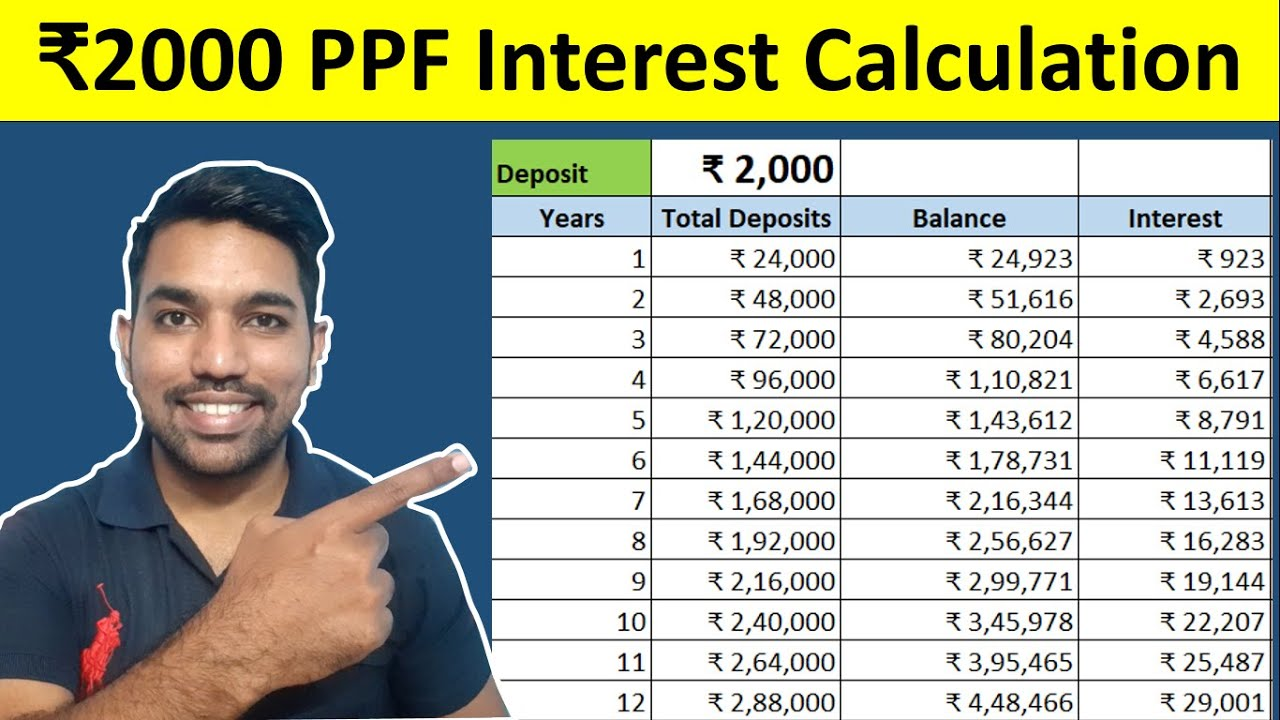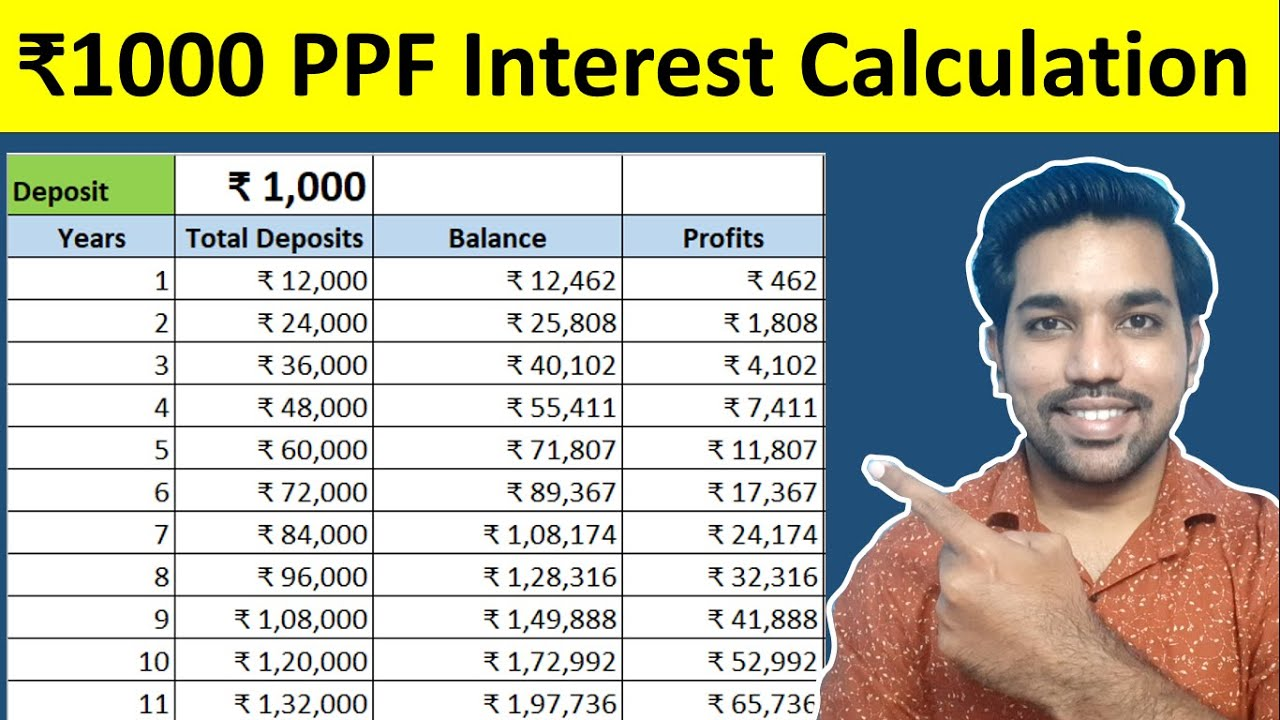# Monthly vs Yearly Deposits in PPF (Public Provident Fund)Many viewers ask an important question on my YouTube channel – whether to deposit if PPF on monthly basis or yearly basis for better returns?

Short answer is – if you can afford yearly deposits than maximum of Rs. 1.5 Lakh on or before 5th day of April will earn you maximum interest in PPF throughout the financial year. You can also deposit less than this maximum amount, (Rs. 500 minimum in FY) as lump sum amount on or before 5th April to get maximum interest out of your investments instead of monthly deposits.

This is only when you want to earn maximum interest on your given deposits. If you want to progress slowly towards your 15 year goal and doesn’t want to aggressively take maximum interest from PPF, than you can also deposit small amounts on monthly basis after dividing your investment corpus into 12 months.

Watch below video to understand how monthly interest is calculated in PPF:

## Rs. 2000 monthly Deposits in PPF Video:Watch more Videos on YouTube Channel

Let us now see some features and benefits of PPF.

## PPF Features and Benefits

• PPF full form is Public Provident Fund, which is a government backed saving scheme with lock-in period of 15 years
• You can claim maximum of Rs. 1.5 Lakh as deduction under Section 80C with old tax regime to save income tax with PPF
• Minimum deposits you can make in PPF in financial year is Rs. 500, to keep the account active
• Maximum of Rs. 1.5 Lakh can be deposited in PPF in every financial year, as a lump sum amount or on monthly basis after dividing this amount based on your choice
• Currently, the latest interest rate in PPF for quarter July 2023 to September 2023 is 7.1%. This interest rate is subjected to be reviewed every quarter by government of India
• Check latest Post Office Interest Rates for other schemes
• It is interesting to note than interest is calculated on your PPF balance every month and is credited on yearly basis on 31st March – end of financial year
• For interest calculation, balance at the end of 5th day of every month is considered for interest calculation for than month
• If you make deposit on or after 6th day of month, than this deposit will start earning interest from next month onwards
• Since interest rate is subjected to change on quarterly basis, the latest interest rate is divided by 12 to compute the monthly interest on your PPF balance using below formula
``Monthly Interest = Yearly Interest Rate / 12 * Balance (on 5th day of month) / 100``

ALSO READ: Section 80C Deductions List

Let us now see some examples with yearly and monthly deposits in PPF.

## Yearly Deposits Example

Let us assume we need to make maximum deposit of Rs. 1.5 lakh in financial year in PPF. You can deposit any amount lower than this based on your affordability and investment strategies.

If you open account in April month and deposit Rs. 1.5 Lakh on or before 5th day, April month interest will be calculated using above formula as:

``````Monthly Interest = Yearly Interest Rate / 12 * Balance (on 5th day of month) / 100
Monthly Interest = 7.1% / 12 * Rs. 1,50,000 / 100
Monthly Interest = Rs. 887.50``````

So you earn interest of Rs. 887.50 for the month of April. You can open PPF account in any month and deposit same or different amount, there is no such hard rule or much impact on interest calculation portion.

Now since we have already made maximum deposit in the start of financial year, we will not make any more deposits until March of next year. So due to no change in Balance, we will earn same interest amount every month up to March of next year.

So your total yearly interest will be:

``Yearly Interest = Rs. 887.50 * 12 = Rs. 10,650``

You earn Rs. 10,650 after adding all monthly interest from this Balance in your PPF account. In 2nd financial year, this interest amount is added to your Balance to earn more interest due to yearly compounding in PPF.

Below is the screenshot of this calculation using our PPF Calculator Online:

So Rs. 10,650 is the total interest you earn in first financial year if you make yearly deposit of Rs. 1.5 Lakh at the start of the financial year.

Let us now compute the yearly interest you can get based on your monthly deposits using example below.

More Recommended Books Here

## Monthly Deposits Example

We’ll take the same example of Rs. 1.5 lakh as the total deposits to be made in PPF, but this time we will divide it into 12 parts – Rs. 12,500 every month to be deposited between April to March financial year cycle.

So for April month interest calculation of Rs. 12,500 deposit on or before 5th day of April, below is the monthly interest calculation:

``````Monthly Interest = Yearly Interest Rate / 12 * Balance (on 5th day of month) / 100
Monthly Interest = 7.1% / 12 * Rs. 12,500 / 100
Monthly Interest = Rs. 73.96``````

This time the April month interest is less since the deposit made by you is less.

Similarly, you will make another deposit of Rs. 12,500 on or before 5th day of May month:

``````Monthly Interest = Yearly Interest Rate / 12 * Balance (on 5th day of month) / 100
Monthly Interest = 7.1% / 12 * Rs. 25,000 / 100
Monthly Interest = Rs. 147.92``````

Now since you have made another deposit of Rs. 12,500 in May, your updated Balance is Rs. 25,000 on which interest is calculated.

So May month interest is Rs. 147.92.

Similarly on other month’s deposits, you will earn interest on updated balance every month. Below is the screenshot from PPF calculator for this calculation:

So as seen above in monthly deposits, your total interest amount after first financial year is Rs. 5769.

And for Yearly deposits, the total interest in first financial year was Rs. 10,650. That’s a difference of Rs. 4881 as interest amount in first financial year.

So in this way you are getting more interest amount on yearly deposits, but while doing monthly deposits, you have the extra amount to be used in case of any emergency if your emergency funds are not ready yet.

So it depends on you how you would want to invest with what amount in your PPF account.

Below is the interest earned in every year based on monthly and yearly deposits in PPF:

Please note that we have used 7.1% annual interest rate throughout the tenure of PPF account and in above examples for simplicity of calculations. In reality, the interest rates might go up or down which might change the actual interest amounts to some extent.

You can use the PPF calculator to know the exact interest amounts by providing the latest interest rates.

## Rs. 1000 Monthly Deposits in PPF

Watch below video to know the total interest that can be earned with Rs. 1000 deposits in PPF:Watch more Excel Videos Here

## Conclusion

So making yearly deposits will give you more interest compared to monthly deposits in your PPF account, but only if you can afford to make lump sum investments instead of diving it into multiple months.

It is not bad to make monthly deposits as well, since it will give you peace of mind to some extent, and you can use the remaining amount in case of any emergency.

## You can Donate Too!

Found this Helpful? DONATE any amount to see more useful Content. Scan below QR code using any UPI App!

UPI ID: abhilashgupta8149-1@okhdfcbank

Verify that you are “Paying Abhilash Gupta” before making the transaction so that it reaches me. It makes my Day 🙂

Thank you for Donating. Stay Tuned!

## Income Tax Calculator App – FinCalC

For Income Tax Calculation on your mobile device, you can Download my Android App “FinCalC” which I have developed for you to make your income tax calculation easy.

### What you can do with this mobile App?

• Calculate Income Tax for new FY 2023-24 and previous FY 2022-23
• Enter estimated Investments to check income tax with Old and New Tax Regime
• Save income tax details and track regularly
• Know how much to invest more to save income tax
• More calculators including PPF, SIP returns, Savings account interest and lot more